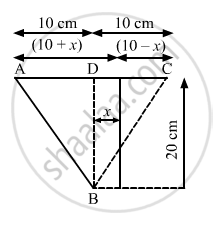Department of Pre-University Education, KarnatakaPUC Karnataka Science Class 11
Advertisement Remove all ads

# The Two Sources of Sound, S1 and S2, Emitting Waves of Equal Wavelength 20.0 Cm, Are Placed with a Separation of 20.0 Cm Between Them. a Detector Can Be Moved on a Line Parallel to S1 S2 - Physics

Sum

The two sources of sound, S1 and S2, emitting waves of equal wavelength 20.0 cm, are placed with a separation of 20.0 cm between them. A detector can be moved on a line parallel to S1 S2 and at a distance of 20.0 cm from it. Initially, the detector is equidistant from the two sources. Assuming that the waves emitted by the sources are in detector should be shifted to detect a minimum of sound.

Advertisement Remove all ads

#### Solution

Given:
Wavelength of sound wave λ = 20 cm
Separation between the two sources AC = 20cm
Distance of detector from source BD = 20 cmIf the detector is moved through a distance x, then the path difference of the sound waves from sources A and C reaching B is given by:
Path difference = AB$-$BC
=$\sqrt{\left( 20 \right)^2 + \left( 10 + x \right)^2} - \sqrt{\left( 20 \right)^2 + \left( 10 - x \right)^2}$

To hear the minimum, this path difference should be equal to :

$\frac{\left( 2n + 1 \right)\lambda}{2}$=$\frac{\lambda}{2}$= 10 cm

So,

$\sqrt{\left( 20 \right)^2 + \left( 10 + x \right)^2} - \sqrt{\left( 20 \right)^2 + \left( 10 - x \right)^2}$= 10
On solving, we get, x = 12.6 cm.
Hence, the detector should be shifted by a distance of 12.6 cm.

Concept: Wave Motion
Is there an error in this question or solution?
Advertisement Remove all ads

#### APPEARS IN

HC Verma Class 11, 12 Concepts of Physics 1
Chapter 16 Sound Waves
Q 30 | Page 354
Advertisement Remove all ads
Advertisement Remove all ads
Share
Notifications

View all notifications

Forgot password?